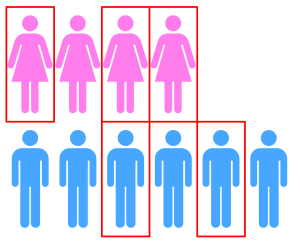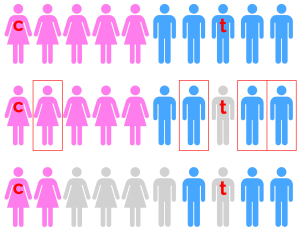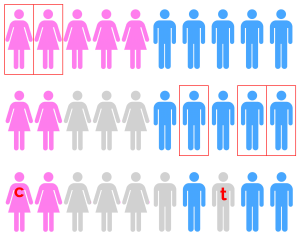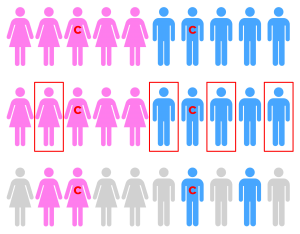# Proof by storytelling

Sit in your favourite chair and do away with those tedious algebraic proofsThere are many equivalent ways of defining the binomial coefficients $\binom{n}{r}$ (pronounced ‘$n$ choose $r$’).  In this article, though, $\binom{n}{r}$ is defined simply as the number of ways of choosing a subset of $r$ things from a set of $n$ things.  Note that this definition does not give us a way to calculate $\binom{n}{r}$; and, if you already know how to evaluate the binomial coefficients, you should put the formula out of your mind and let yourself be surprised by the beautiful way in which identities can be proved without resorting to bashing out messy fractions of factorials.

The following proofs are known as proofs by double counting, or, perhaps more endearingly, story proofs.  The idea is that you can prove that two expressions are equal by telling two different stories that describe the size of the same set in two different ways.  First, a simple example:
$\sum_{k=0}^{n} \binom{n}{k} = 2^n. \tag{1}$
This identity is commonly proved by induction, but there is an elegant proof that anybody, equipped with the above definition of $\binom{n}{r}$, can understand.

Let’s tell a story. There are $n$ light switches on a wall. In how many ways can they be configured? The left-hand side of (1) counts this: it starts by counting the number of ways in which zero light switches can be chosen to be on, then does the same for one, then two, and so on, until it counts the number of ways in which all $n$ light switches can be turned on. But the right-hand side counts the same sum: each of the $n$ light switches could be either on or off, so for each light switch there are two choices and so the total number of configurations is $2^n$. Thus the left-hand side and the right-hand side give the size of the same set, and (1) is true.

Another identity that is susceptible to a story proof is Vandermonde’s convolution:
\begin{equation}
\sum_{k=0}^{r} \binom{m}{k} \binom{n}{r-k} = \binom{m+n}{r}. \tag{2}
\end{equation}
Proving it algebraically requires you to play around with the product of two sums, which, as you can imagine, is both messy and also not particularly insightful.A choice of $r$ people from $m$ men and $n$ women.

Having said that, (2) does admit a very nice proof through storytelling. The clue as to what story to tell is the right-hand side. The binomial coefficient $\binom{m+n}{r}$ suggests that we ought to consider choosing $r$ things from $m+n$ things.  Consider a set of $m+n$ people—$m$ males and $n$ females—from which we would like to select a committee of $r$ people. The right-hand side counts this in an obvious way: it neglects gender and just chooses $r$ people from $m+n$. The left-hand side is less obvious. It first counts how many ways we can choose zero males and $r$ females ($\binom{m}{0} \binom{n}{r}$), then one male and $r-1$ females ($\binom{m}{1} \binom{n}{r-1}$), all the way up to $r$ males and no females ($\binom{m}{r} \binom{n}{0}$). In this way, it counts every possible committee. So the left-hand side and the right-hand side count the same set and so are equal. Not only is the story proof more elegant than the algebraic proof, it is actually shorter, and gives some intuition as to why the identity is true!

Both examples so far are well known, but this method extends to situations that are less common. Let’s think about Vandermonde’s convolution in the case where $m=n=r$ and, instead of committees, think about teams. For this it’s necessary to note that $\binom{n}{r} = \binom{n}{n-r}$. You can convince yourself that this is so by considering that the number of ways of choosing $r$ things from $n$ things is the same as the number of ways of choosing $r$ things to exclude from $n$ things.  In this case, Vandermonde’s convolution becomes
\begin{equation}
\sum_{k=0}^{n} \binom{n}{k} ^2 = \binom{2n}{n}. \tag{3}
\end{equation}
How can we interpret this?

Well, the right-hand side is the number of ways of choosing a team of $n$ people from $2n$ people, $n$ of whom are male and $n$ of whom are female. The left-hand side is the number of ways of choosing a team of $n$ people comprised of zero males, then one male, then two males, and so on, until we enumerate each possibility. So (3) is true, but Vandermonde had already told us that. What more interesting stories can we tell?A choice of $n$ team members from $n$ men and $n$ women, including a captain.

Well, every team should have a captain: so in how many ways can we do this? Considering the right-hand side first, we can extend it as follows. Choose $n$ people (we can do this in $\binom{2n}{n}$ ways), then choose a captain from them (we can do this in $n$ ways), so the number of ways of choosing a team of $n$ people from $2n$, with a captain, is $n\binom{2n}{n}$. To count all of the possible teams in a different way, let’s consider teams which have $k$ men in them, and then sum over all the possible values of $k$: $0, 1, 2, \dots, n$. Say that my team has $k$ males in it, and that my captain is male. I can choose the men in $\binom{n}{k}$ ways, the women in $\binom{n}{n-k}$ ways, then choose my captain in $k$ ways. Noting that $\binom{n}{k} = \binom{n}{n-k}$, we find that there are $k\binom{n}{k}^2$ teams with $k$ males and a male captain. Similarly, there are $(n-k)\binom{n}{k}^2$ teams with $k$ males and a female captain, and we can rewrite this as $(n-k)\binom{n}{n-k}^2$.  So the total number of teams with $k$ males and a captain of any gender is $k\binom{n}{k}^2 + (n-k)\binom{n}{n-k}^2$. Thus we sum over all values of $k$, recovering the identity:
\begin{gather*}
\sum_{k=0}^{n} \left[ k\binom{n}{k} ^2 + (n-k)\binom{n}{n-k}^2 \right] = n\binom{2n}{n},
\end{gather*}
or, by noting that the second part of the sum is in fact the same as the first part, just in reverse order, we can write this more succinctly as:
\begin{equation}\label{eq:captain}
\sum_{k=0}^{n} 2k\binom{n}{k} ^2 = n\binom{2n}{n}. \tag{4}
\end{equation}

Now that we have seen various applications of telling stories, we are ready for the big league. Consider the following identity:
\begin{equation}\label{eq:scorer}
\sum_{k=0}^n k^{\,2} \binom{n}{k}^2 = n^2\binom{2(n-1)}{n-1}. \tag{5}
\end{equation}
It goes without saying that (5) would be an absolute mess to prove algebraically, but is there a nice story proof? It turns out that yes, there is, and that it is even cleaner than the proof of (4). If you would like to have a go at proving the identity yourself, stop reading now!

Let’s count the number of ways of forming a team of $n$ people from a set of $2n$ people, $n$ of whom are male and $n$ female. The twist is that our team must include a female captain, and that we must also designate a male timekeeper, who must not be on the team. Thus the right-hand side of (5) can easily be interpreted: we first choose one of $n$ females to be captain and put her on the team, then one of $n$ males to be timekeeper. Finally we choose the remaining $n-1$ people we need on the team from the $2(n-1)$ people left.

How does the left-hand side count the same thing?  Consider a team that contains $k$ females. We first choose the $k$ females in $\binom{n}{k}$ ways, then the remaining $n-k$ males in $\binom{n}{n-k}=\binom{n}{k}$ ways. Then we choose a captain from the $k$ females on the team in $k$ ways, and a scorer from the $k$ males not chosen in $k$ ways. Thus the right-hand side is equal to the sum of $k^{\,2}\binom{n}{k}$ over all $k$. So (5) is true.A team made of $n$ members, including a female captain. A male timekeeper, not on the team, is also chosen.A team made up of $k$ females and $n-k$ males, with one of the $k$ chosen females as captain and one of the $k$ males not chosen as timekeeper.

The identity in (5) was proved using the intuition gained from the right-hand side, but what happens if we try to garner information from the left-hand side? It turns out that a completely different story proof is possible. What feeling do we get from the left-hand side? Well, each binomial coefficient is squared, so it seems natural to consider all the possible teams with the same number ($n$) of males and females. That is, we reinterpret the left-hand side as counting the total number of teams with the same number of male members as female members, which also have a designated male and female captain.A team made by choosing a female and male captain, then choosing $n-1$ of the remaining $2(n-1)$ people. Of the $n-1$ people chosen, add the picked females and the unpicked males to the team.

How can we reinterpret the right-hand side to align with this? We first choose a male captain and a female captain, and put both of these people on our team. We can do this in $n^2$ ways. Then we choose half of the remaining people. We can do this in $\binom{2(n-1)}{n-1}$ ways. This choice doesn’t seem to have anything to do with choosing an equal number of males and females, but here’s the trick: from our choice, we generate a new selection by swapping all the males that we’ve chosen for all the males we haven’t chosen, and use that selection instead. A little thought shows that for each selection of half of the remaining people, we get exactly one team with equal numbers of males and females, and vice versa, as shown in the diagram. Thus the number of teams with the same number of males and females as well as a male and female captain is $n^2\binom{2(n-1)}{n-1}$. So we have proved (5) in two different ways, both by telling stories, and both more succinctly than we could have done algebraically!

Now that we have established that story proofs are viable methods for proving identities, perhaps you would like to try your hand at a few identities yourself?

$\sum_{k=2}^n k(k-1)\binom{n}{k} = n(n-1)\times 2^{n-2}$

$\sum_{k=0}^r \binom{n}{k} \binom{n-k}{r-k} = 2^r \binom{n}{r}$

$\sum_{k=0}^n k^{\,2} = \frac{1}{6}n\,(n+1) (2n+1)$

The author would like to thank Dominic Rowland for introducing him to the idea of story proofs, and would like to recommend the book Introduction to Combinatorics, published by the United Kingdom Mathematics Trust (UKMT) and authored by Gerry Leversha and Dominic Rowland, which expands on these techniques.

[Header image used with kind permission from Jane Hissey, janehissey.co.uk]Jack is an undergraduate studying mathematics at Corpus Christi College, University of Cambridge. When he’s not telling stories, Jack can be found volunteering for the UKMT, composing choral music, and playing the organ. [Photo: Heppy Longworth]

## 2 thoughts on “Proof by storytelling”

1. Pingback: Maths and storytelling – Form 12D